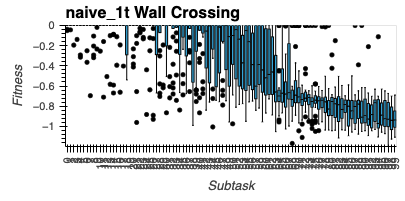# Changing X-Axis Ticks in Holoviews Categorical Axis

I recently ran into the issue of changing how many ticks I want displayed on the x-axis in a holoviews plot. My data ranged from 0 to 100, but it was categorical in the pandas dataframe. Simple solutions don’t work in this case as categorical data can’t have the ticks changed easily in opts. Instead, I found this solution at: https://stackoverflow.com/questions/49460699/bokeh-plot-custom-categorical-tick-labels

Implementing it in my case allowed me to go from:Far too many ticks on the x-axis to be readable. Importance is just noting that the objectives increase from left to right and not so much each individual one.

To this:

Code for the solution is below. Mainly, making the CategoricalAxis not visible and adding in a LinearAxis with FixedTicker did the trick.

```import holoviews as hv

from bokeh.models import CategoricalTicker, FixedTicker, Ticker
from bokeh.models.formatters import NumeralTickFormatter
from bokeh.util.compiler import TypeScript
from bokeh.plotting import show
from bokeh.io import output_notebook, export_png
from bokeh.models.axes import LinearAxis
from bokeh.layouts import gridplot

def format_x_axis(p):
# Remove default axis
p.xaxis.visible = False

ticker = FixedTicker(ticks=[0,50,100])
xaxis = LinearAxis(ticker=ticker)
xaxis.axis_label = "Objective"

return p

# Create a box plot to show how turning performs
def create_boxwhisker_custom_ticks(df, group_col, y_col, title, ylim=(-1.2,0)):
boxwhisker = hv.BoxWhisker(df, [group_col], y_col).opts(width=300,xrotation = 90,ylim=ylim, title=title)
return boxwhisker

plots = []
plot_width = 400
plot_height= 200

for t in wt_treatments:
t += "_wt"
filtered_df = wt_best_last_gen_per_rep_full_results_df[wt_best_last_gen_per_rep_full_results_df.Trt == t]